Courses

# Test: Communication Systems - 5

## 25 Questions MCQ Test Communication Theory | Test: Communication Systems - 5

Description
This mock test of Test: Communication Systems - 5 for Electronics and Communication Engineering (ECE) helps you for every Electronics and Communication Engineering (ECE) entrance exam. This contains 25 Multiple Choice Questions for Electronics and Communication Engineering (ECE) Test: Communication Systems - 5 (mcq) to study with solutions a complete question bank. The solved questions answers in this Test: Communication Systems - 5 quiz give you a good mix of easy questions and tough questions. Electronics and Communication Engineering (ECE) students definitely take this Test: Communication Systems - 5 exercise for a better result in the exam. You can find other Test: Communication Systems - 5 extra questions, long questions & short questions for Electronics and Communication Engineering (ECE) on EduRev as well by searching above.
QUESTION: 1

Solution:

24 = 16.

QUESTION: 2

### When modulation index of an AM wave is increased from 0.5 to 1, the transmitted power

Solution: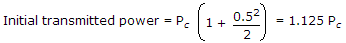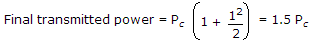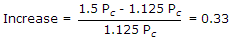.

QUESTION: 3

### Quantizing error occurs in

Solution:

An analog number cannot be converted into an exact digital number.

This is called quantizing error.

QUESTION: 4

In audio cassettes the width of each recording track is

Solution:

Each track is 2.5 mm wide.

QUESTION: 5

The most commonly used transistor amplifier circuit is

Solution:

Common emitter connection has high power gain, and good current and voltage gains.

QUESTION: 6

When energy is fed into open wire transmission line the energy may get dissipated due to

Solution:

All the three cause dissipation of energy as heat.

QUESTION: 7

Fourier series expansion of a real periodic signal with fundamental frequency is given by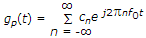It is given that c3 = 3 + j5. then c-3 is

Solution:

For real signal

ck = c*-k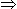3 - i5.

QUESTION: 8

Loudness control gives

Solution:

For depth in sound the low frequency notes, (i.e., bass) need more amplification.

QUESTION: 9

If transmitted power is 75 kW, the field strength at 30 km distance will be about

Solution:

Using the derivation,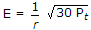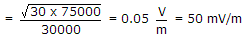.

QUESTION: 10

A mobile telephone has a range of about

Solution:

Mobile telephone has about 50 km range.

QUESTION: 11

In the television system in India, each frame is scanned

Solution:

Each frame is divided into odd and even fields. Each field is scanned 25 times so that each frame is scanned 50 times.

QUESTION: 12

Each kilometer of travel in electromagnetic radiation means a time delay of

Solution:

Since velocity of em waves is 300 m/ms, each kilometer means time delay of 3.3 μs.

QUESTION: 13

A video monitor is exactly similar to TV receiver.

Solution:

Trinitron is a colour picture tube.

QUESTION: 14

A RADAR can be used to

Solution:

Radar is used for all the three purposes.

QUESTION: 15

In single tone AM modulation, the transmission efficiency for m = 1 is

Solution:

Since signal power = 0.5 PC and total power is 1.5 PC efficiency is 33.3%.

QUESTION: 16

AM amplifier having noise figure of 20 dB and available power gain of 15 dB is followed by a mixer circuit having noise figure of 9 dB. The overall noise figure as referred to input in dB is

Solution: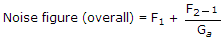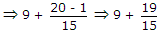.

QUESTION: 17

If the oscillator output is modulated by audio frequencies upto 10 kHz, the frequency range occupied by the side bands will be

Solution:

Sideband frequencies will be fc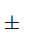fm.

QUESTION: 18

Which one of the following is non-resonant antenna?

Solution:

Rhombic antenna is a non-resonant antenna capable of operating over 3 to 30 MHz range.

QUESTION: 19

The efficiency of using a binary system for selection of 1 out of 13 equally probable events is

Solution:

log2 13 = 3.7 therefore, number of bits = 4.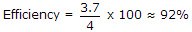.

QUESTION: 20

The most suitable method for detecting a modulated signal g(t) = (3 to 6 cos 2pfmt) cos 2pfct is

Solution:

Because it is an AM envelope.

QUESTION: 21

The time required for horizontal blanking is 16% of that for each horizontal line. If horizontal time is 63.5 μs, the horizontal blanking time for each line is about

Solution: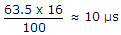.

QUESTION: 22

A message signal with bandwidth 10 kHz is lower sideband SSB modulated with carrier frequency fc1 = 106 Hz. The resulting signal is then passed though a narrow band frequency modulator with carrier frequency fc2 = 109 Hz. The bandwidth of the output would be

Solution:

Bandwidth = 2Δf + 2B

But for NBFM, B < 1BW ≈ 2fm2fc12 x 106 Hz.

QUESTION: 23

When the number of quantising levels is 16 in PCM, the number of pulses in a code group will be

Solution:

2n = Ln = 4.

QUESTION: 24

In a PCM system, if the code word length is increased from 6 to 8 bits, the signal to quantization noise ratio improves by the factor.

Solution:

Given n1 = 6, n2 = 8,

then (SNR)Q = 22(n2 - n1)16.

QUESTION: 25

For 10 bit PCM system, the signal to quantization noise ratio is 62 dB. If the number of bits is increased by 2, then the signal to quantization noise ratio will

Solution: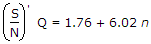= (SNR)Q + 6.02 x 2(SNE)Q + 12 dB.